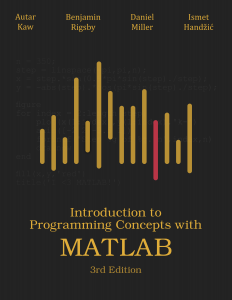# Introduction to Programming Concepts with MATLAB: Third EditionIntroduction to Programming Concepts with MATLAB
Third Edition
By Autar Kaw, Benjamin Rigsby, Daniel Miller and Ismet Handzic

Purchase Book

Preview Textbook

Author Biographies

This book is intended for an introductory course in programming in STEM (science, technology, engineering, and mathematics) fields while using MATLAB as the programming language. MATLAB is a popular computational software package used in universities and industries alike.

This textbook differentiates itself from others in two specific ways.

1. The textbook is suitable for the many engineering departments throughout the nation that no longer teach a 3-credit hour programming course. They weave programming and mathematical software packages such as MATLAB in courses such as Foundations of Engineering, Freshmen Design, Modeling of Systems, Engineering Analysis, Numerical Methods, etc. This book is highly suitable for such audiences. To achieve these goals and make the access far-reaching, we have been deliberate in keeping the lessons short in length so that instructors can easily choose the course content in a modular way.
2. The textbook is a stand-alone resource for learning programming where the lectures complement the textbook rather than vice versa. This is because of the reason above where in-classroom time is truncated, and therefore students need to be more self-taught. For this reason, we have been meticulous when selecting and organizing the textbook content to include fundamental and application programming problems that prepare students well for other problems they will solve in academia and industry.

The book has nine modules which have been each broken down by lessons. There are 42 lessons in all and depending on the learning outcomes of the course, an instructor can choose to assign only necessary lessons.

Modules 1-3 focus on MATLAB and programming basics like the MATLAB program interface, programming variables, different types of data, debugging, plotting, and applications to science and engineering problems. In Module 4, we show the use of MATLAB for basic mathematical procedures learned in the engineering courses including nonlinear equations, integration, differentiation, simultaneous linear equations, interpolation, regression, and ordinary differential equations.

In Modules 5-8, the user is introduced to basic programming concepts of conditional statements, repetition (loops), and custom functions.

In Module 9, program input/output is shown with writing to and reading from external files as well as navigating directories with MATLAB.

Important appendices include a primer on matrix algebra, a collection of mini-projects, and a introduction to animating plots in MATLAB. Appendix A provides a primer on matrix algebra. Appendix B contains a set of mini-projects. Appendix C demonstrates how to make animated plots in MATLAB.

Each lesson contains screenshots of actual MATLAB programs that are used to help illustrate the concepts presented. More than 120 complete programs are shown throughout this book to demonstrate to the reader how to use programming concepts. The book is written in a USA-Today style question-answer format for a quick grasp of the concepts.

The purpose of this book is to provide the reader with a firm basic understanding of MATLAB syntax and fundamental programming concepts. Each lesson contains MATLAB programs that are used to help illustrate the concepts presented. By no means do the authors claim to present every MATLAB command, function, application, or programming concept in existence.

The textbook, written by four authors led by Autar Kaw (2012 US Professor of the Year) has several unique features, has a companion website for quick reference, and this and earlier editions have been adopted by several departments.